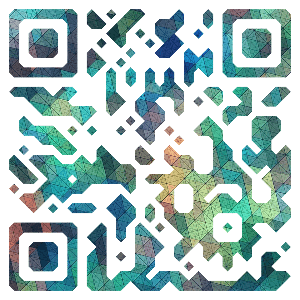# 决策树基本流程

## 总体流程

“分而治之”（divide-and-conquer）

• 自根至叶的递归过程
• 在每个中间结点寻找一个“划分”（split or test）属性

## 三种停止条件

• 当前结点包含的样本全属于同一类别，无需划分
• 当前属性集为空，或是所有样本在所有属性上取值相同（类别不同），无法划分
• 当前结点包含的样本集合为空，不能划分

# 划分选择

## ID3

ID3(Iterative Dichotomiser)是以信息增益为准则来选择划分属性的决策树学习算法，迭代二分器的简称

“信息熵”是度量样本集合“纯度”最常用的一种指标，假定当前样本集合 $D$ 中的第$k$类样本所占比例 $p_k(k=1,2,...,|y|)$，则信息熵定义为：

$Ent(D)=-\sum_{k=1}^{|y|}p_klog_2p_k \tag{1}$

$Ent(D)$ 的值越小，则 $D$ 的纯度越高。

1. （等概情况）如果 $p_k$ 有 m 类，每个 $p_k$ 都为 $\frac{1}{m}$ 的话，信息熵取得一个最大值 $log_2{|y|}$，我们是最不确定的，即纯度最低。
2. （确定情况）如果 $p=0或1$，则信息熵为 0，纯度最高

• 所谓离散属性 $a$ 就是这个特征（或属性）有可以穷尽的离散取值，如下面的属性集合 $A=\lbrace a^1（长相）,a^2 （年龄）,a^3（收入） \rbrace$，长相你可以定义为帅或者不帅（只有 2 个有限取值）
• $D^V$ 是以某属性对 $D$ 进行划分的子集，如“长相”则可得到 2 个子集，记为 $D^1(长相=帅)$$D^2(长相=不帅)$
• $D^1$ 里则有 1 号 xxx，2 号 xxx 等备选对象

$Gain(D,a)=Ent(D)-\sum_{v=1}^V \frac{|D^v|}{|D|}Ent(D^v) \tag{2}$

$Ent(D) =-\sum_{k=1}^2p_k log_2 p_k =-(\frac{8}{17}log_2\frac{8}{17}+\frac{9}{17}log_2\frac{9}{17}) =0.998$

$a^1(色泽)，a^2(根蒂)，a^3(敲声)，a^4(纹理)，a^5(脐部)，a^6(触感)$

\begin{aligned}Ent(D^1)=-(\frac {3}{6}log_2\frac{3}{6}+\frac {3}{6}log_2\frac{3}{6})=1.000\\Ent(D^2)=-(\frac {4}{6}log_2\frac{4}{6}+\frac {2}{6}log_2\frac{2}{6})=0.918\\Ent(D^3)=-(\frac {1}{5}log_2\frac{1}{5}+\frac {4}{5}log_2\frac{4}{5})=0.722 \end{aligned}

\begin{aligned}Gain(D,色泽)&=Ent(D)-\sum_{v=1}^3\frac{|D^v|}{|D|}Ent(D^v) \\&=0.998-(\frac{6}{17}\times1.000+\frac{6}{17}\times0.918+\frac{5}{17}\times0.722)\\&=0.109 \end{aligned}

\begin{aligned} &Gain(D,根蒂)=0.143 \qquad Gain(D,敲声)=0.141\\ &Gain(D,纹理)=0.381 \qquad Gain(D,脐部)=0.289\\ &Gain(D,触感)=0.006 \end{aligned}

\begin{aligned} &Gain(D^1,色泽)=0.043 \qquad Gain(D^1,根蒂)=0.458\\ &Gain(D^1,敲声)=0.331 \qquad Gain(D^1,脐部)=0.458\\ &Gain(D^1,触感)=0.458 \end{aligned}

“根蒂”、“脐部”、“触感”3 个属性均取得了最大的信息增益，可任选其中之一作为划分属性。这里我们选择“根蒂”作为划分属性，然后递归的去完成这样的过程，对每个分支结点进行上述操作。最终得到的决策树如下图所示。

## C4.5

$Gain\_ratio(D,a)=\frac{Gain(D,a)}{Ⅳ(a)}$

$Ⅳ(a)=-\sum_{v=1}^V \frac{|D^v|}{D}log_2\frac{|D^v|}{D}$

\begin{aligned} & Ⅳ(触感)=0.874(V=2)\quad Ⅳ(色泽)=1.580(V=3) \quad Ⅳ(根蒂)=1.402(V=3)\\ & Ⅳ(敲声)=0.973(V=3) \quad Ⅳ(纹理)=1.447(V=3) \quad Ⅳ(脐部)=1.549(V=3)\\ & Ⅳ(编号)=4.088(V=17) \end{aligned}

## CART

CART(Classification and Regression Tree) 是一种著名的二分类决策树学习算法，分类和回归任务都可用。CARRT 决策树使用“基尼指数”（Gini index）来选择划分属性。

1. 是一种不等性度量；
2. 通常用来度量收入不平衡，可以用来度量任何不均匀分布；
3. 是介于 0~1 之间的数，0 完全相等，1 完全不相等；
4. 总体内包含的类别越杂乱，GINI 指数就越大（跟熵的概念很相似）

\begin{aligned} Gini(D)&=\sum_{k=1}^{|y|}\sum_{k'\not= k}p_kp_{k'} \qquad p_k'=1-p_k\\ &=1-\sum_{k=1}^{|y|}p_k^2 \end{aligned}

$Gini\_index(D,a)=\sum_{v=1}^V\frac{|D^v|}{|D|}Gini(D^v)$

\begin{aligned} Gini\_index(D,纹理) &= \frac{9}{17}×Gini(D_1)+\frac{5}{17}×Gini(D_2)+\frac{3}{17}×Gini(D_3) \\ & = \frac{9}{17}×\{1-[(\frac{7}{9})^2+(\frac{2}{9})^2]\}+\frac{5}{17}×\{1-[(\frac{1}{5})^2+(\frac{4}{5})^2]\}+\frac{3}{17}×[1-(0^2+1^2)] \\ & = 0.27712 \end{aligned}

# 参考资料

• CART 算法中的基尼指数
• 周志华-《机器学习》微信支付宝